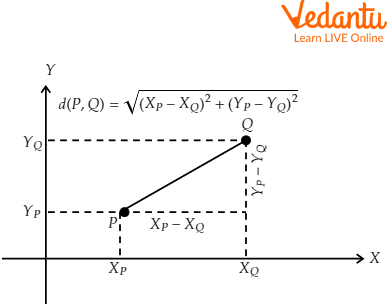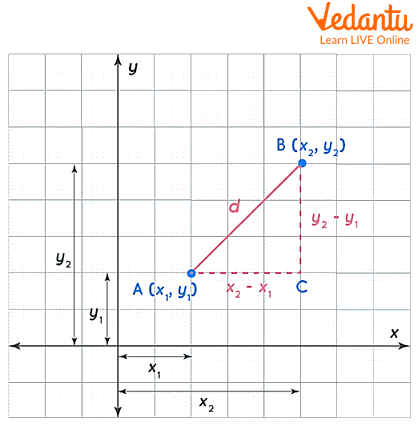Courses
Courses for Kids
Free study material
Offline Centres
More

# Euclidean Distance FormulaLast updated date: 24th Nov 2023
Total views: 57.6k
Views today: 1.57k## What is Euclidean Distance? An Introduction

The Euclidean distance, a positive number, represents the spacing between two points in a space where Euclid's geometry's axioms and theorems are true. The distance between points A and B in a Euclidean space is the length of the line segment AB belonging to the only line that passes through these points.

The space we perceive and where we human beings move is a three-dimensional space (3-D), where the axioms and theorems of Euclid's geometry are fulfilled. Two-dimensional subspaces (flats) and one-dimensional subspaces (lines) are contained in this space.

Euclidean spaces can be one-dimensional (1-D), two-dimensional (2-D), and three-dimensional (3-D).

## Euclidean Distance in One Dimension

The oriented line (OX) connects all points in the one-dimensional space X, and the direction from O to X is positive.Euclidean distance in 1 D

To locate the points on said line, the Cartesian system is used, which consists of assigning a number to each point on the line.

The Euclidean distance d(A, B) between points A and B, located on a line, is defined as the square root of the square of the differences of their X coordinates:

$d(A,B) = \sqrt {({{({X_B} - {X_A})}^2})}$

This definition guarantees that: The distance between two points is always a positive quantity. And that the distance between A and B is equal to the distance between B and A.

## Euclidean Distance in Two Dimensions

Two-dimensional Euclidean space is a plane. The points of a Euclidean plane satisfy the axioms of Euclidean geometry, for example:

• Only one line connects two points.

• Three points on the plane form a triangle whose internal angles always add up to 180º.

• The square of the hypotenuse in a right triangle equals the sum of the squares of its legs.

• A point has X and Y coordinates in two dimensions.

For instance, the coordinates of a point P are (XP, YP), whereas those of a point Q are (XQ, YQ).

The Euclidean distance between the points P and Q is defined with the following formula:

$d(P,Q) = \sqrt {[{{({X_Q} - {X_P})}^2} + {{({Y_Q} - {Y_P})}^2}]}$

It should be noted that this formula is equivalent to the Pythagorean theorem, as shown in Figure 2.The distance between two points P and Q of the plane fulfils the Pythagorean theorem

## The Euclidean Distance Formula Derivation

To derive the Euclidean distance formula, let us consider two points, A (x1, y1) and B (x1, y2) and assume that d is the distance between them. Join A and B by a line segment. We create a right-angled triangle using AB as the hypotenuse to arrive at the formula. To do this, we construct lines from points A and B that meet at point C, as illustrated below.Derivation of euclidean distance formula

The Pythagorean theorem will now be applied to the triangle ABC. Then comes,

$A{B^2} = A{C^2} + B{C^2}$

${d^2} = {\left( {{x_2} - {x_1}} \right)^2} + {\left( {{y_2} - {y_1}} \right)^2}$

Taking the square root on both sides,

$d = \sqrt {{{\left( {{x_2} - {x_1}} \right)}^2} + {{\left( {{y_2} - {y_1}} \right)}^2}}$

Hence the Euclidean distance formula is derived.

Let’s now see some solved Euclidean distance examples.

## Solved Examples

Example 1: Find the Euclidean distance between points (2,4) and (-2, -4).

Solution: Given points are (x1, y1) = (2,4) and (x2, y2) = (-2, -4)

Use Euclidean distance formula,

$d = \sqrt {\left[ {{{\left( {{x_2} - {x_1}} \right)}^2} + {{\left( {{y_2} - {y_1}} \right)}^2}} \right]}$

$d = \sqrt {\left[ {{{\left( { - 2 - 2} \right)}^2} + {{\left( { - 4 - 4} \right)}^2}} \right]}$

$d = \sqrt {{{\left( { - 4} \right)}^2} + {{\left( { - 8} \right)}^2}}$

$d = \sqrt {16 + 64}$

$d = \sqrt {80}$

$d = 4\sqrt 5$ units

Example 2: Find the distance between points (1,1) and (5,4).

Sol: Given points are (x1, y1) = (1,1) and (x2, y2) = (5,5)

Use Euclidean distance formula,

$d = \sqrt {\left[ {{{\left( {{x_2} - {x_1}} \right)}^2} + {{\left( {{y_2} - {y_1}} \right)}^2}} \right]}$

$d = \sqrt {\left[ {{{\left( {5 - 1} \right)}^2} + {{\left( {5 - 1} \right)}^2}} \right]}$

$d = \sqrt {{4^2} + {4^2}}$

$d = \sqrt {16 + 16}$

$d = \sqrt {32} \,units$

## Key Features about Euclidean Distance

• The Euclidean distance in mathematics is the length of a line segment connecting two points in Euclidean space.

• The distance between the two locations should be calculated from the length of the line segment that connects them.

• Since it could be calculated from the points' cartesian coordinates using the Pythagorean Theorem, it is frequently referred to as the Pythagorean distance.

• These designations are drawn from the ancient Greek mathematicians Euclid and Pythagoras, even though Euclid did not characterise distances as numbers and the connection between the Pythagorean theorem and distance calculation was not understood until the 18th century.

## Summary

The Euclid distance formula for 1 dimensional is the absolute value of the difference x-coordinates of the points. The Euclid distance of 1 dimensional is a line segment. The y-coordinate of the points is zero.

The Euclid distance formula for 2 dimensional is $d = \sqrt {{{\left( {{x_2} - {x_1}} \right)}^2} + {{\left( {{y_2} - {y_1}} \right)}^2}}$.

## Practice Questions

Q 1: Find the distance between points (1,2) and (3,5) using the Euclidean distance formula.

Ans: $\sqrt {13}$ units.

Q 2: Find the distance between points A (2,8) and B (5,8).

Ans: 3 units.

## FAQs on Euclidean Distance Formula

1: What is the distance formula for collinear points?

If the sum of the distances between any two pairs of points is equal to the distance between the remaining pair of points, then three points are collinear. This is known as the distance formula for collinear points.

2: Why do we use Euclidean distance?

The Euclidean distance measures the separation between two points with real values. When determining the distance between two rows of data with numerical values, such as floating point or integer values, you are most likely to utilise the Euclidean distance.

3: Why does the distance formula work?

Finding the hypotenuse of a right triangle is the same as finding the distance between two separate points on a plane. According to the distance formula, the separation between any two locations on a plane is determined by taking the square root of the sum of the squares of the rise and run.